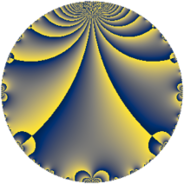Properties

 Label 8.3.dLevel $8$ Weight $3$ Character orbit 8.d Rep. character $\chi_{8}(3,\cdot)$ Character field $\Q$ Dimension $1$ Newform subspaces $1$ Sturm bound $3$ Trace bound $0$

Related objects

Defining parameters

 Level: $$N$$ $$=$$ $$8 = 2^{3}$$ Weight: $$k$$ $$=$$ $$3$$ Character orbit: $$[\chi]$$ $$=$$ 8.d (of order $$2$$ and degree $$1$$) Character conductor: $$\operatorname{cond}(\chi)$$ $$=$$ $$8$$ Character field: $$\Q$$ Newform subspaces: $$1$$ Sturm bound: $$3$$ Trace bound: $$0$$

Dimensions

The following table gives the dimensions of various subspaces of $$M_{3}(8, [\chi])$$.

Total New Old
Modular forms 3 3 0
Cusp forms 1 1 0
Eisenstein series 2 2 0

Trace form

 $$q - 2 q^{2} - 2 q^{3} + 4 q^{4} + 4 q^{6} - 8 q^{8} - 5 q^{9} + O(q^{10})$$ $$q - 2 q^{2} - 2 q^{3} + 4 q^{4} + 4 q^{6} - 8 q^{8} - 5 q^{9} + 14 q^{11} - 8 q^{12} + 16 q^{16} + 2 q^{17} + 10 q^{18} - 34 q^{19} - 28 q^{22} + 16 q^{24} + 25 q^{25} + 28 q^{27} - 32 q^{32} - 28 q^{33} - 4 q^{34} - 20 q^{36} + 68 q^{38} - 46 q^{41} + 14 q^{43} + 56 q^{44} - 32 q^{48} + 49 q^{49} - 50 q^{50} - 4 q^{51} - 56 q^{54} + 68 q^{57} - 82 q^{59} + 64 q^{64} + 56 q^{66} + 62 q^{67} + 8 q^{68} + 40 q^{72} - 142 q^{73} - 50 q^{75} - 136 q^{76} - 11 q^{81} + 92 q^{82} + 158 q^{83} - 28 q^{86} - 112 q^{88} + 146 q^{89} + 64 q^{96} - 94 q^{97} - 98 q^{98} - 70 q^{99} + O(q^{100})$$

Decomposition of $$S_{3}^{\mathrm{new}}(8, [\chi])$$ into newform subspaces

Label Dim. $$A$$ Field CM Traces $q$-expansion
$a_{2}$ $a_{3}$ $a_{5}$ $a_{7}$
8.3.d.a $1$ $0.218$ $$\Q$$ $$\Q(\sqrt{-2})$$ $$-2$$ $$-2$$ $$0$$ $$0$$ $$q-2q^{2}-2q^{3}+4q^{4}+4q^{6}-8q^{8}+\cdots$$